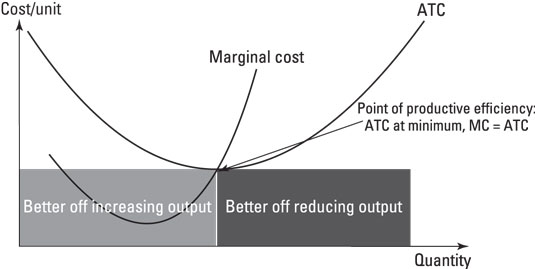##### Microeconomics For Dummies, USA EditionProductive efficiency is satisfied when a firm can't possibly produce another unit of output without increasing proportionately more the quantity of inputs needed to produce that unit of output. It's met when the firm is producing at the minimum of the average cost curve, where marginal cost (MC) equals average total cost (ATC). (Sometimes you will see ATC as just AC, or average cost. They mean the same thing.)

Why is that? At the minimum of the average total cost curve, economies of scale are exhausted, and production at this level yields the lowest per unit cost. The firm is producing an output level at the lowest possible cost. If a firm expands production beyond that point, it incurs a marginal cost higher than the average cost, and the per-unit cost of output increases.

A firm maximizes profits by producing where marginal revenue equals marginal cost, or MR = MC. If this occurs at the same output level where MC = ATC, then profit maximization leads to productive efficiency.

You can use the concept of productive efficiency to tell you a lot about how a market is operating. One application is in considering how society should treat natural monopolies — those companies that yield sufficient economies of scale relative to the size of the total market that they're unlikely to ever face a direct competitor.

One thing economists notice is that these companies tend to operate inefficiently; that is, that they don't tend to operate at the lowest possible cost (and that consumers are consequently hurt by this, as inefficiencies get pushed on to the consumer in the form of lower quality or quantity and/or higher prices).

The figure summarizes productive efficiency: The two shaded areas reveal how the firm can become better off by making itself more productive.Productive efficiency.